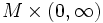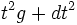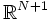# Riemannian cone

Given a Riemannian manifold$(M,g)$, the Riemannian cone of$M$ is defined as the manifold$M \times (0,\infty)$ equipped with the cone metric:$t^2 g + dt^2$
Equivalently, if we actually embed the Riemannian manifold in Euclidean space$\R^N$, then this is the Riemannian manifold given by a cone in$\R^{N+1}$ whose section is$M$ (as embedded in$\R^N$).